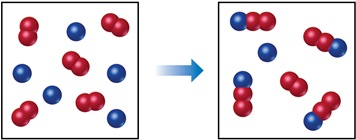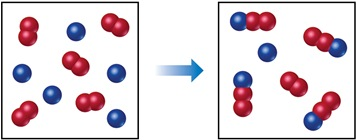# Problem: The following diagram represents a reaction shown going to completion. Each molecule in the diagram represents 0.1 mol, and the volume of the box is 1.0 L.Assuming that all of the molecules are in the gas phase, calculate n, the change in the number of gas molecules that accompanies the reaction.

###### FREE Expert Solution

We're being asked to calculate n, the change in the number of gas molecules that accompanies the reaction.

Given: diagram represents 0.1 mol

A = red

B = blue100% (355 ratings)###### Problem Details
The following diagram represents a reaction shown going to completion. Each molecule in the diagram represents 0.1 mol, and the volume of the box is 1.0 L.Assuming that all of the molecules are in the gas phase, calculate n, the change in the number of gas molecules that accompanies the reaction.

Frequently Asked Questions

What scientific concept do you need to know in order to solve this problem?

Our tutors have indicated that to solve this problem you will need to apply the Chemical Equilibrium concept. You can view video lessons to learn Chemical Equilibrium. Or if you need more Chemical Equilibrium practice, you can also practice Chemical Equilibrium practice problems.

What professor is this problem relevant for?

Based on our data, we think this problem is relevant for Professor Kuntz's class at UNC.